# Reinforced Neighborhood Selection Guided Multi-Relational Graph Neural Networks 阅读笔记

## 1. 介绍

• 第一个基于多关系图的任务驱动GNN框架，充分利用关系采样、消息传递、度量学习和强化学习来指导不同关系内部和之间的邻居选择。
• 一种灵活的邻域选择框架，该框架采用增强的关系感知邻域选择器和标签感知神经相似性邻域度量。
• 一种递归的、可扩展的强化学习框架，通过对不同规模的图形或任务的可估计深度和宽度来学习优化的过滤阈值。
• 第一次从不同关系的重要性角度研究多关系GNN的可解释性。

## 2、背景和概述

### 2.1 问题定义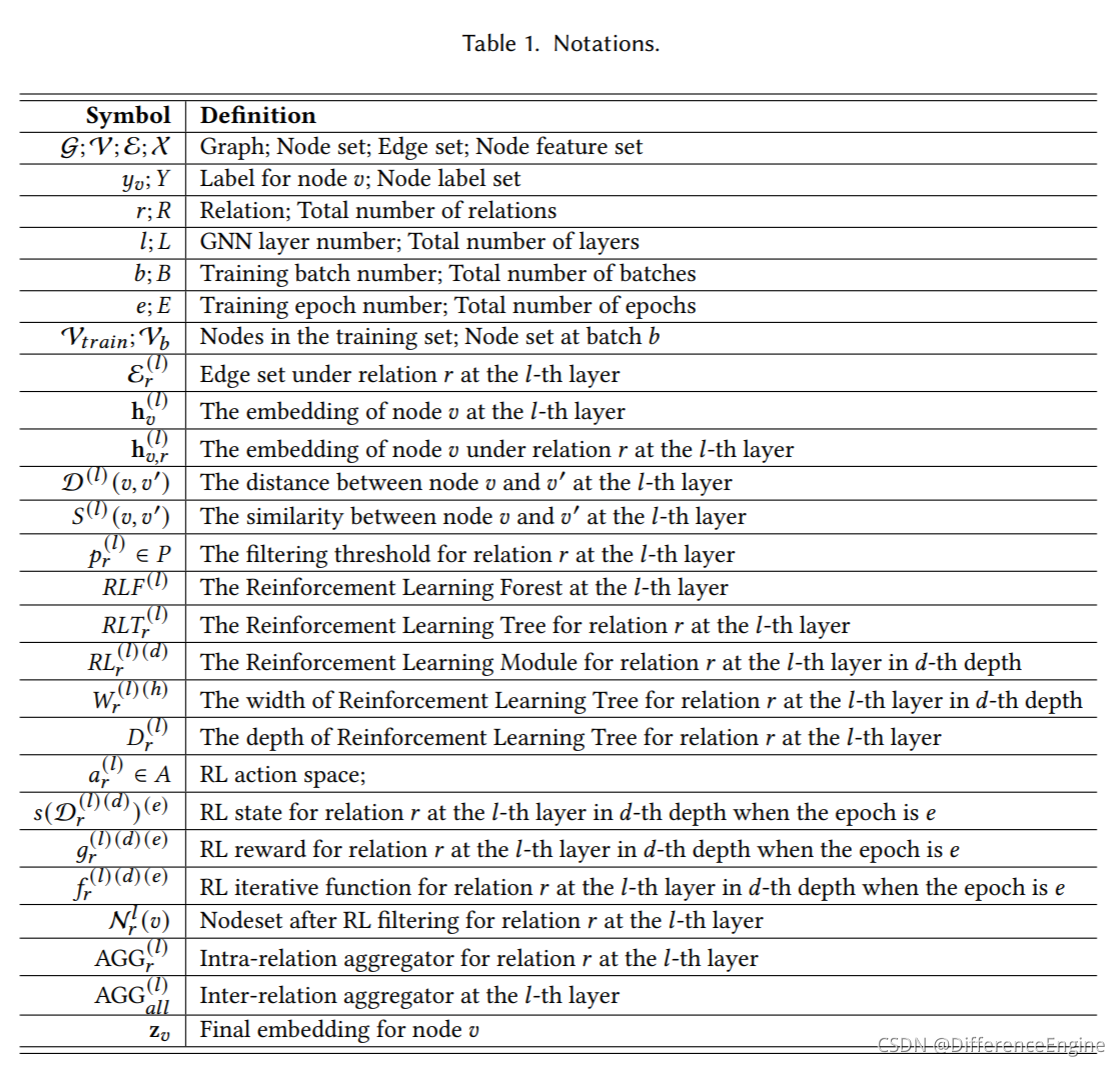• 垃圾邮件审查检测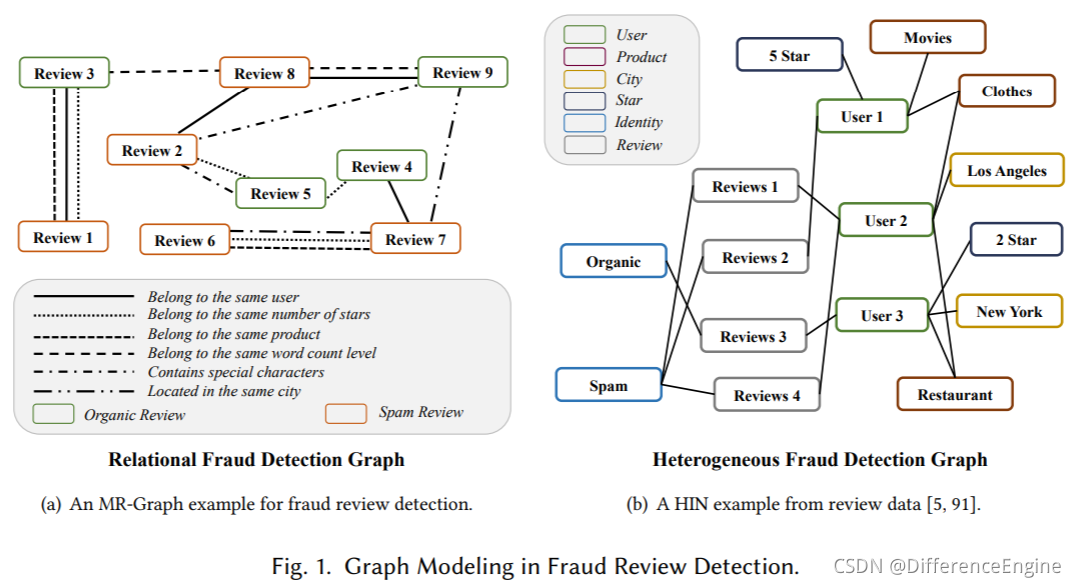• 疾病诊断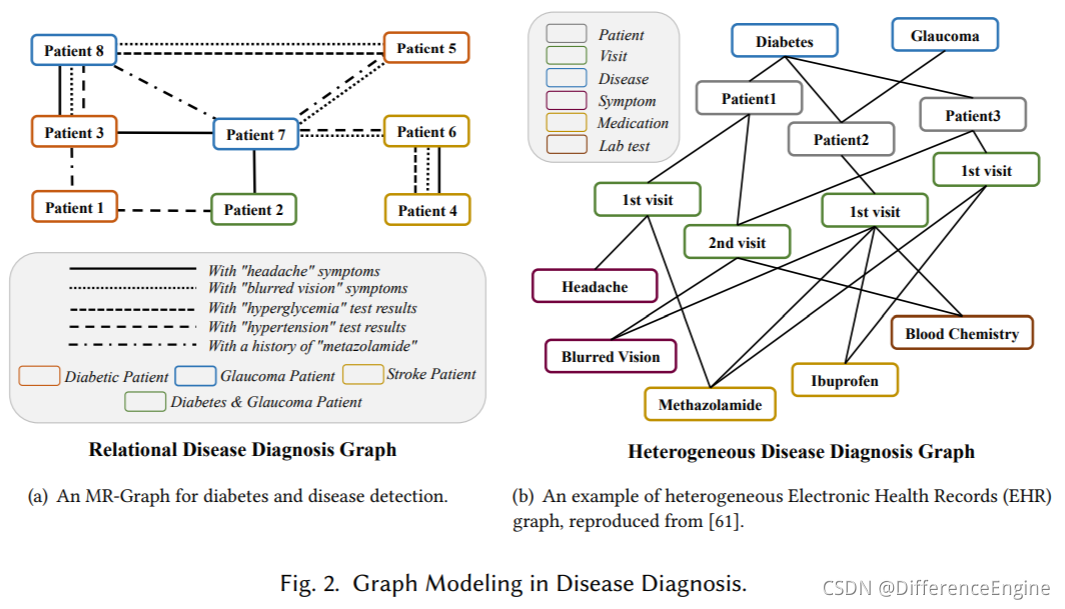h v ( l ) = σ ( h v ( l − 1 ) ⊕ A G G ( l ) ( { h v ′ , r ( l − 1 ) : ( v , v ′ ) ∈ E r ( l ) } ∣ r = 1 R ) ) , (1) \mathbf{h}_{v}^{(l)}=\sigma\left(\mathbf{h}_{v}^{(l-1)} \oplus A G G^{(l)}\left(\left.\left\{\mathbf{h}_{v^{\prime}, r}^{(l-1)}:\left(v, v^{\prime}\right) \in \mathcal{E}_{r}^{(l)}\right\}\right|_{r=1} ^{R}\right)\right),\tag{1}

## 三、方法论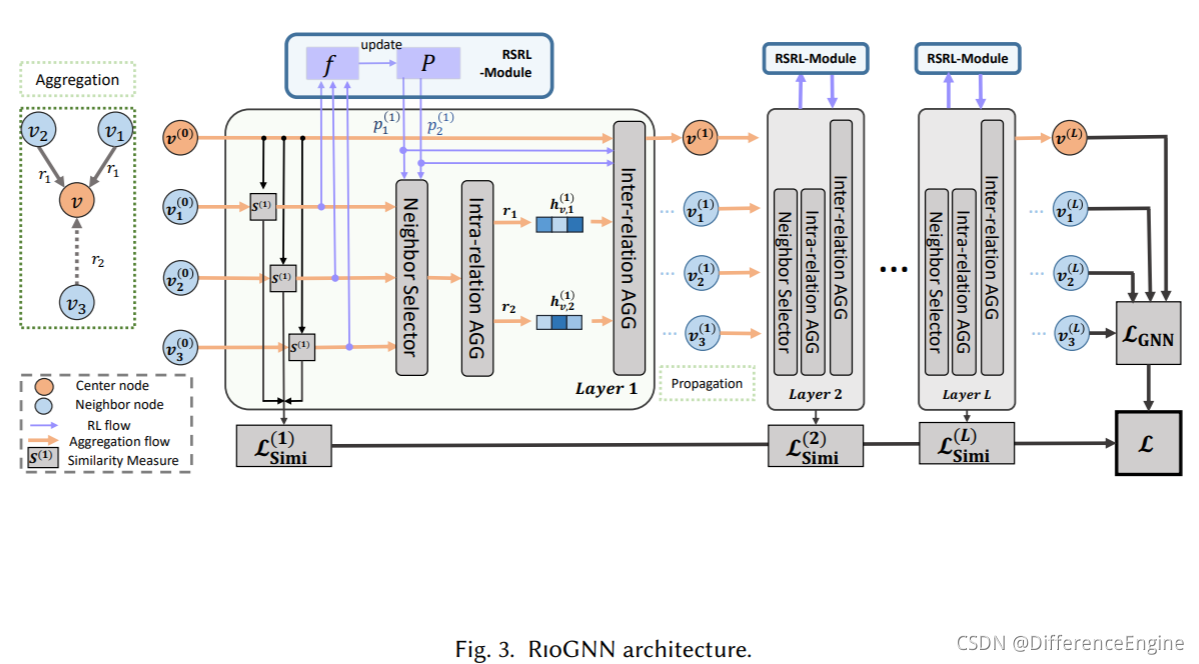### 3.1 标签感知神经相似性度量

D ( l ) ( v , v ′ ) = ∥ σ ( F C N ( l ) h v ( l − 1 ) ) − σ ( F C N ( l ) h v ′ ( l − 1 ) ) ∥ 1 . (2) \mathcal{D}^{(l)}\left(v, v^{\prime}\right)=\left\|\sigma\left(F C N^{(l)} \mathbf{h}_{v}^{(l-1)}\right)-\sigma\left(F C N^{(l)} \mathbf{h}_{v^{\prime}}^{(l-1)}\right)\right\|_{1}.\tag2

S ( l ) ( v , v ′ ) = 1 − D ( l ) ( v , v ′ ) . (3) S^{(l)}\left(v, v^{\prime}\right)=1-\mathcal{D}^{(l)}\left(v, v^{\prime}\right).\tag3

L Simi  ( l ) = ∑ v ∈ V − log ⁡ ( y v ⋅ σ ( F C N ( l ) ( h v ( l ) ) ) ) . (4) \mathcal{L}_{\text {Simi }}^{(l)}=\sum_{v \in \mathcal{V}}-\log \left(y_{v} \cdot \sigma\left(F C N^{(l)}\left(\mathbf{h}_{v}^{(l)}\right)\right)\right).\tag4

L Simi  = ∑ l = 1 L L Simi  ( l ) . (5) \mathcal{L}_{\text {Simi }}=\sum_{l=1}^{L} \mathcal{L}_{\text {Simi }}^{(l)}.\tag5

### 3.2 相似性感知自适应邻居选择器

#### 3.2.2 RSRL框架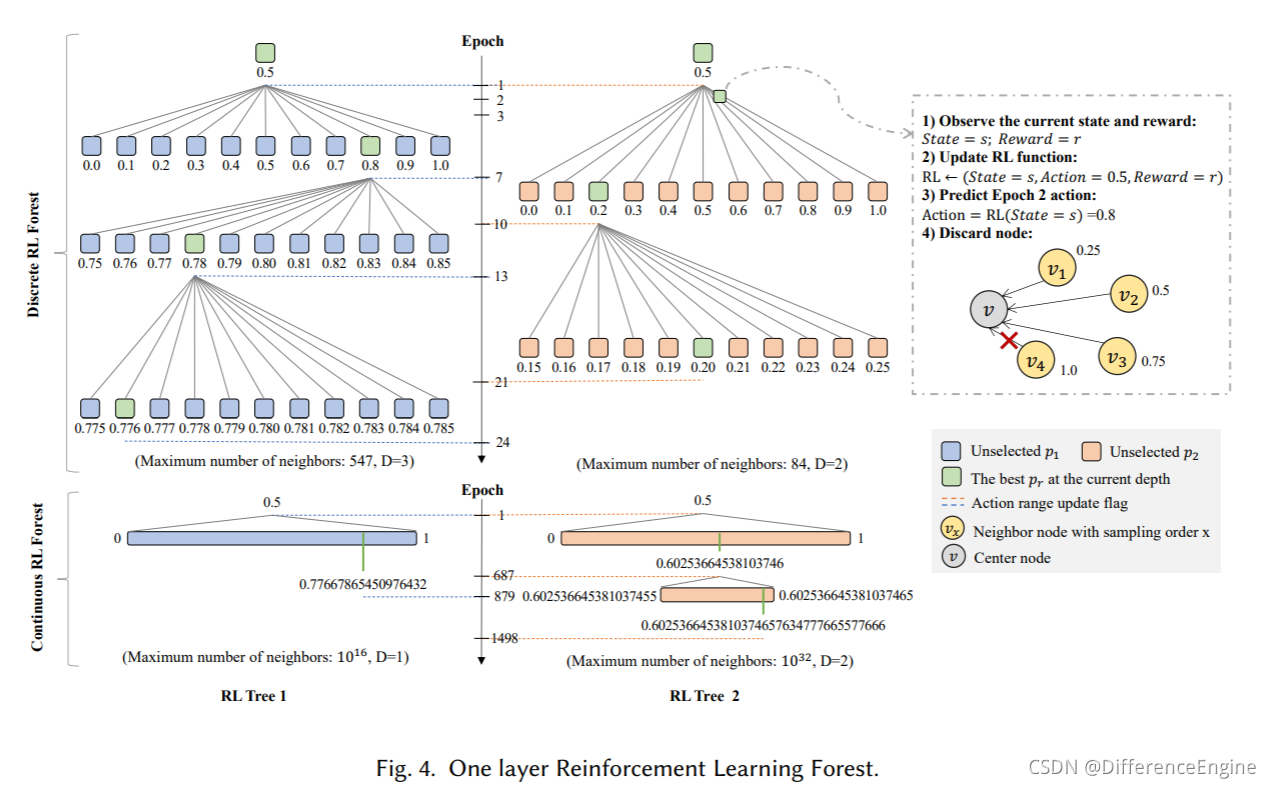R L F ( l ) = { R L T r ( l ) } ∣ r = 1 R = { { R L r ( l ) ( d ) } ∣ d = 1 D r ( l ) } ∣ r = 1 R , (6) R L F^{(l)}=\left.\left\{R L T_{r}^{(l)}\right\}\right|_{r=1} ^{R}=\left.\left\{\left.\left\{R L_{r}^{(l)(d)}\right\}\right|_{d=1} ^{D_{r}^{(l)}}\right\}\right|_{r=1} ^{R},\tag6
R L F ( l ) RLF^{(l)}实际上是指在第l层获得最佳关系滤波阈值组合的过程。每个关系独立地以自适应的深度D r ( l ) = ⌈ log ⁡ α k r ⌉ D_{r}^{(l)}=\left\lceil\log _{\alpha} k_{r}\right\rceil和宽度W r ( l ) ( d ) = 1 α d W_{r}^{(l)(d)}=\frac{1}{\alpha^{d}}构造RL树R L F ( l ) RLF^{(l)}α \alpha是深度优先和宽度优先的权重参数，k r ( l ) k^{(l)}_r是关系 r 中包含在节点中的最大邻居数。R L F ( l ) RLF^{(l)}执行强化学习R L r ( l ) ( d ) RL^{(l)(d)}_r在每个深度以精度W r ( l ) ( d ) W_{r}^{(l)(d)}过滤阈值。在第l层，R L F ( l ) RLF^{(l)} 通过多次 RL 递归获取精度高于前一关系深度 r 的邻居节点的最佳过滤阈值 p r ( l ) ( d ) p_r^{(l)(d)}，直到在深度 D r ( l ) D_r^{(l)} 处找到最大精度要求的阈值。R L F ( l ) RLF^{(l)} 递归过程表示为：
p r ( l ) ( d ) ⟵ R L r ( l ) ( d ) { p r ( l ) ( d − 1 ) − W r ( l ) ( d ) 2 , p r ( l ) ( d − 1 ) + W r ( l ) ( d ) 2 } . (7) p_{r}^{(l)(d)} \stackrel{R L_{r}^{(l)(d)}}{\longleftarrow}\left\{p_{r}^{(l)(d-1)}-\frac{W_{r}^{(l)(d)}}{2}, p_{r}^{(l)(d-1)}+\frac{W_{r}^{(l)(d)}}{2}\right\}.\tag7

• 动作 当关系 r 在第 l 层的深度 d 时，我们通过收集所有动作a r ( l ) ( d ) ∈ { p r ( l ) ( d − 1 ) − W r ( l ) ( d ) 2 , p r ( l ) ( d − 1 ) + W r ( l ) ( d ) 2 } a_{r}^{(l)(d)} \in\left\{p_{r}^{(l)(d-1)}-\frac{W_{r}^{(l)(d)}}{2}, p_{r}^{(l)(d-1)}+\frac{W_{r}^{(l)(d)}}{2}\right\}来定义R L r ( l ) ( d ) RL^{(l)(d)}_r的动作空间 A。离散方案 D-RL 将动作空间平均划分为 α \alpha 个离散动作。 在连续方案C-RL中，第l深度的动作空间是一个宽度为W r ( l ) ( d ) W_{r}^{(l)(d)}的连续浮点数。两种动作空间的兼容性可以有效适应多种强化学习算法。 由于对中小规模数据集的过滤阈值精度要求不高，动作离散化可以在满足基本精度要求的同时减少动作探索的次数，保证高效访问高性能区域。 对于具有大规模邻居的数据集，大量满足高精度要求的离散动作会影响学习效果。 我们建议将过滤阈值推广到连续动作空间，以通过多次减少空间范围来提高大规模数据集的准确性。

• 状态 由于无法将 GNN 的分类损失直接感知为环境状态，我们通过标签感知的距离度量（式（2））计算每个 epoch 的平均节点距离作为状态。 在第 e 个 epoch 的第 l 层，第 d 个深度的关系 r 的状态 s 为：

s r ( l ) ( d ) ( e ) = ∑ ( v , v ′ ) ∈ E r ( l ) ( d ) ( e ) D ( l ) ( v , v ′ ) ( e ) ∣ E r ( l ) ( d ) ( e ) ∣ , (8) s_{r}^{(l)(d)(e)}=\frac{\sum_{\left(v, v^{\prime}\right) \in \mathcal{E}_{r}^{(l)(d)(e)} \mathcal{D}^{(l)}\left(v, v^{\prime}\right)^{(e)}}}{\left|\mathcal{E}_{r}^{(l)(d)(e)}\right|},\tag8

• 奖励 对于每个关系，目标是确定一个过滤阈值 p r ( l ) ( d ) p_r^{(l)(d)}，以便选择的邻居节点和中心节点尽可能接近。 因此，我们使用相似度（公式（3））作为奖励函数中的决定性因素。 在第 e 个 epoch 的第 l 层，关系 r 与第 d 个深度的奖励 g 为：

g r ( l ) ( d ) ( e ) = τ ⋅ ( ∑ ( v , v ′ ) ∈ E r ( l ) ( d ) ( e ) S ( l ) ( v , v ′ ) ( e ) ∣ E r ( l ) ( d ) ( e ) ∣ ) , (9) g_{r}^{(l)(d)(e)}=\tau \cdot\left(\frac{\sum_{\left(v, v^{\prime}\right) \in \mathcal{E}_{r}^{(l)(d)(e)} \mathcal{S}^{(l)}\left(v, v^{\prime}\right)^{(e)}}}{\left|\mathcal{E}_{r}^{(l)(d)(e)}\right|}\right),\tag9

• 迭代与终止 在开始每个 epoch 之前，RL 观察上一个动作a r ( l ) ( d ) ( e − 1 ) a_{r}^{(l)(d)(e-1)} 的 epoch 之后环境的状态 s r ( l ) ( d ) ( e ) s_{r}^{(l)(d)(e)}，并获得奖励 g r ( l ) ( d ) ( e − 1 ) g_{r}^{(l)(d)(e-1)}。然后用它们来更新迭代函数（广义上指的是策略迭代的函数或强化的值迭代过程） 学习）f f。 每个R L r ( l ) ( d ) RL^{(l)(d)}_r的迭代函数如下：

f r ( l ) ( d ) ← s r ( l ) ( d ) ( e − 1 ) , s r ( l ) ( d ) ( e ) , a r ( l ) ( d ) ( e − 1 ) , g r ( l ) ( d ) ( e − 1 ) . (10) f_{r}^{(l)(d)} \leftarrow s_{r}^{(l)(d)(e-1)}, s_{r}^{(l)(d)(e)}, a_{r}^{(l)(d)(e-1)}, g_{r}^{(l)(d)(e-1)}.\tag{10}

p r ( l ) ( d ) ( e ) = a r ( l ) ( d ) ( e ) = f r ( l ) ( d ) ( s r ( l ) ( d ) ( e ) ) . (11) p_{r}^{(l)(d)(e)}=a_{r}^{(l)(d)(e)}=f_{r}^{(l)(d)}\left(s_{r}^{(l)(d)(e)}\right).\tag{11}

{ { p r ( l ) ( d ) ( i ) − p r ( l ) ( d ) ( i − 1 ) = 0 } ∣ i = e − 1 e D − R l ,  where  e > 2. { ∣ p r ( l ) ( d ) ( i ) − p r ( l ) ( d ) ( i − 1 ) ∣ < W r ( l ) ( d ) } ∣ i = e − 1 e C − R L ,  where  e > 2. (12) \begin{cases}\left.\left\{p_{r}^{(l)(d)(i)}-p_{r}^{(l)(d)(i-1)}=0\right\}\right|_{i=e-1} ^{e} & D-R l, \text { where } e>2 .\\ \left.\left\{\left|p_{r}^{(l)(d)(i)}-p_{r}^{(l)(d)(i-1)}\right|<W_{r}^{(l)(d)}\right\}\right|_{i=e-1} ^{e} & C-R L, \text { where } e>2.\end{cases}\tag{12}

### 3.3 关系感知加权邻居聚合器

h v , r ( l ) = ReLU ⁡ ( A G G r ( l ) ( { ⊕ h v ′ ( l − 1 ) : v ′ ∈ N r l ( v ) } ) ) , (13) \mathbf{h}_{v, r}^{(l)}=\operatorname{ReLU}\left(A G G_{r}^{(l)}\left(\left\{\oplus \mathbf{h}_{v^{\prime}}^{(l-1)}: v^{\prime} \in \mathcal{N}_{r}^{l}(v)\right\}\right)\right),\tag{13}

h v ( l ) = Re ⁡ L U ( h v ( l − 1 ) ⊕ A G G ( l ) ( { ⊕ ( p r ( l ) ⋅ h 0 , r ( l ) ) } ∣ r = 1 R ) ) , (14) \mathbf{h}_{v}^{(l)}=\operatorname{Re} L U\left(\mathbf{h}_{v}^{(l-1)} \oplus A G G^{(l)}\left(\left.\left\{\oplus\left(p_{r}^{(l)} \cdot \mathbf{h}_{0, r}^{(l)}\right)\right\}\right|_{r=1} ^{R}\right)\right),\tag{14}

### 3.4 整合

L G N N = ∑ v ∈ V − log ⁡ ( y v ⋅ σ ( M L P ( l ) ( z v ) ) ) . (15) \mathcal{L}_{G N N}=\sum_{v \in \mathcal{V}}-\log \left(y_{v} \cdot \sigma\left(M L P^{(l)}\left(\mathbf{z}_{v}\right)\right)\right).\tag{15}

L RIOGNN  = L G N N + λ l ∑ l = 1 L L Simi  ( l ) + λ ∗ ∥ Θ ∥ 2 , (16) \mathcal{L}_{\text {RIOGNN }}=\mathcal{L}_{G N N}+\lambda_{l} \sum_{l=1}^{L} \mathcal{L}_{\text {Simi }}^{(l)}+\lambda_{*}\|\Theta\|_{2},\tag{16}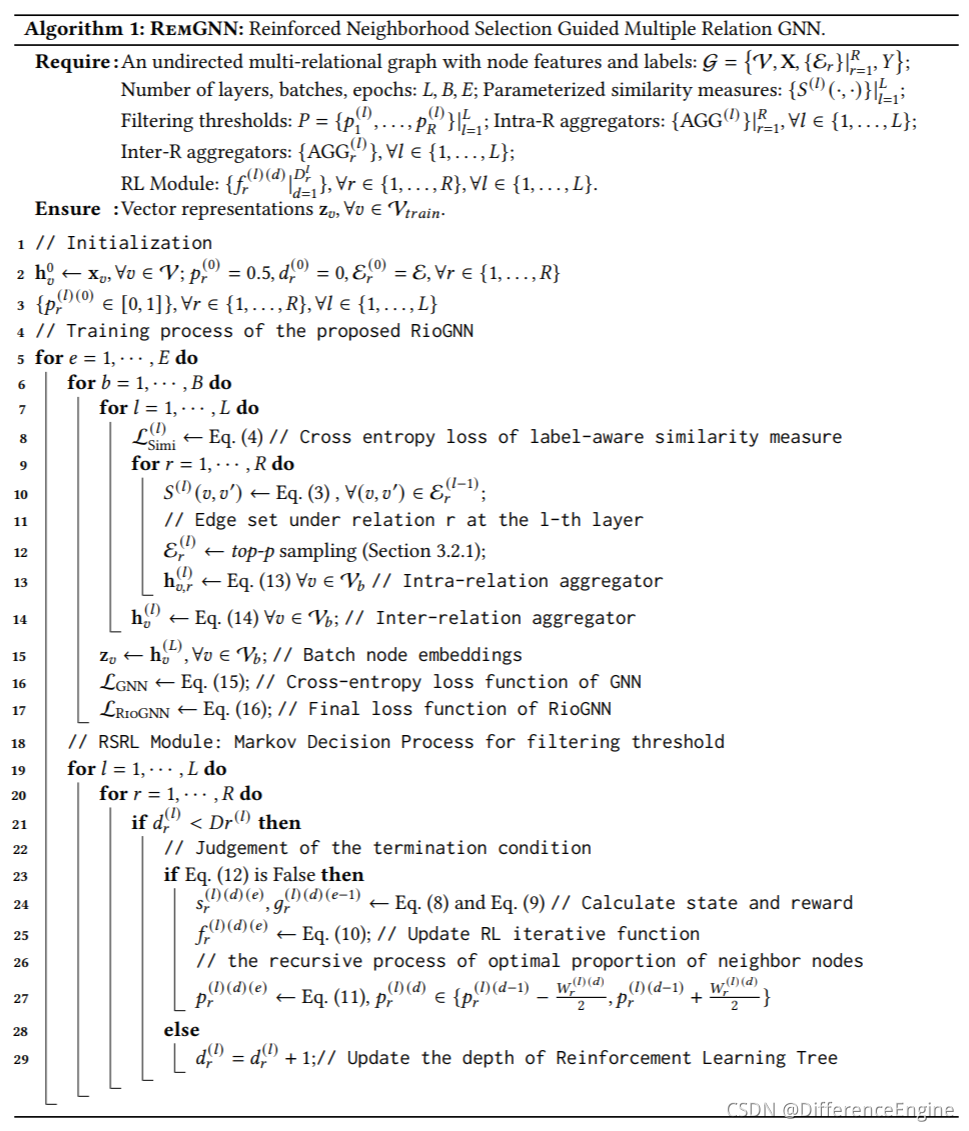RioGNN的时间复杂度 算法1的总体时间复杂度是O ( ∣ E ∣ ⋅ max ⁡ ( { α log ⁡ α k r } ∣ r = 1 R ) ) O\left(|\mathcal{E}| \cdot \max \left(\left.\left\{\alpha \log _{\alpha} k_{r}\right\}\right|_{r=1} ^{R}\right)\right)，其中∣ E ∣ |\mathcal{E}|是边的数量，α \alpha是深度优先和宽度优先的权重参数，k r k_r是与r相关的节点中包含的最大邻居数。这里，O ( ∣ E ∣ ) O(|\mathcal{E}|)是一个epoch的时间复杂度。具体来说，相似性度量（算法 1 中的第 10 行）和聚合（算法 1 中的第 13-15 行）总共需要 O ( ∣ E ∣ d + ∣ V ∣ ( k ˉ d + d ) ) = O ( ∣ E ∣ ) O(|\mathcal{E}| d+|\mathcal{V}|(\bar{k} d+d))=O(|\mathcal{E}|)，其中k ˉ \bar{k}是平均节点度数∣ V ∣ |\mathcal{V}|是节点数量。RSRL模块（算法1中的第21-29行）采用O ( ∣ E ∣ d ) = O ( ∣ E ∣ ) O(|\mathcal{E}| d)=O(|\mathcal{E}|)每个交叉熵损失函数（算法1中的第8行和第16行）取O ( ∣ V ∣ ) O(|\mathcal{V}|)另外，epoch数受强化学习的动作空间影响，达到收敛所需的epoch数与几个关系max ⁡ ( { α log ⁡ α k r } ∣ r = 1 R ) \max \left(\left.\left\{\alpha \log _{\alpha} k_{r}\right\}\right|_{r=1} ^{R}\right)中需求最大的动作空间有关。

## 四、实验组织

• Q 1 : Q1:我们如何在不同的场景中构建多关系图（第4.2节）？
• Q 2 : Q2:RioGNN在欺诈检测任务中的有效性、效率和可解释性（第5.1节）。
• Q 3 : Q3:RioGNN在疾病检测任务中的有效性、效率和可解释性（第5.2节）。
• Q 4 : Q4:不同的任务需求如何匹配通用RSRL框架（第5.3节）？
• Q 5 : Q5:我们的模型在集群任务和归纳学习中表现如何（第5.2.1节、第5.1.1节和第5.4节）？
• Q 6 : Q6:讨论超参数敏感性和对模型的影响（第5.5节）。

### 4.2 数据集与图构建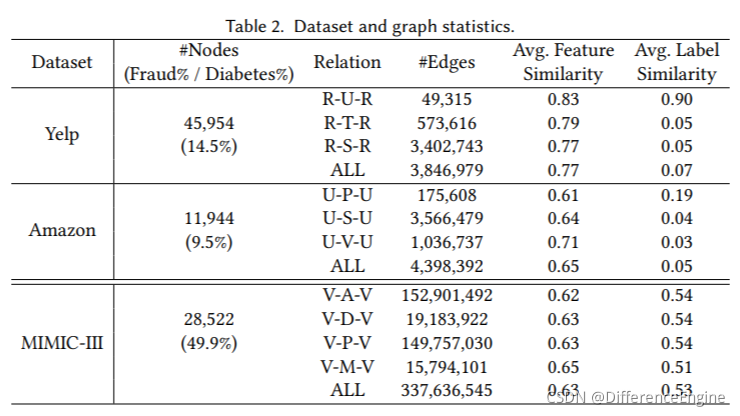#### 4.2.1 欺诈检测任务

YELP: 收集自美国最大的商业评论网站 Yelp.com 发布的内部数据集。我们使用  收集和使用的 YelpChi 数据集的一个子集。该子集包含 45,954 条用户对芝加哥地区酒店和餐馆的评论。评论已被 Yelp 过滤（垃圾邮件）和推荐（合法）。数据集除了包含用户和产品之间的关系信息外，还包含各种元数据，包括评论的文本内容、时间戳和星级。我们使用了32个人工特征，包括所有产品评论的排名顺序、与产品平均值的绝对评分偏差、是否是用户唯一的评论、所有大写单词的百分比、大写字母的百分比、评论的长度、第一人称代词的比例，感叹句的比例，以及中使用的主词比例，客观词比例等作为Yelp数据集的原始节点特征。对于特定的关系，由于之前的研究 [69,82] 已经表明意见欺诈者（即垃圾邮件发送者）在用户、产品、评论文本和时间方面是相互关联的，我们使用评论作为图中的节点并设计了三种关系：

• R-U-R：它连接同一用户发布的评论。
• R-T-R：它连接同一个月发布的同一产品下的两个评论。
• R-S-R：它连接同一产品下具有相同星级（1-5星级）的评论。

Amazon: 是亚马逊产品数据集的子集 。亚马逊数据集包含超过 34,000 条消费者评论，我们从中提取了乐器类别下的 11,949 条产品评论。此外，与类似，我们将有用投票率大于 80% 的用户标记为良性实体，将有用投票率小于 20% 的用户标记为欺诈实体。在节点特征选择方面，我们使用了25个人工特征，包括评分产品数量、用户名长度、用户给出的每个评分等级的数量和比例、正面和负面评论的比例、用户的评分,用户获得的有用和无用票总数,有用票和无用票的比例,以及平均值,有用和无用票的中位数,最小和最大有用和无用票数,用户之间的天数 中作为亚马逊数据集的原始节点函数使用的第一个和最后一个评分、相同的日期指示符、评论文本情绪等。对于具体的关系，我们为多关系图设计了三种关系，如下所示：

• U-P-U：它连接用户至少查看一个相同的产品。
• U-S-V：它连接一周内至少有一个相同星级的用户。
• U-V-U：它将所有用户中互评文本相似度最高的5%（由TF-IDF衡量）的用户连接起来。

#### 4.2.2 糖尿病诊断任务

MIMIC-III: 是一个公开可用的数据集 [25,42]，包含 46,520 名重症监护病房 (ICU) 患者超过 11 年的健康记录。我们总共提取了 28,522 次患者就诊，每条记录包含年龄、诊断、微生物学、程序、语料库等。我们使用  收集和使用的 MIMIC-III 数据集的一个子集，并在此基础上为我们的任务构建多关系图。对于每个患者和访问，都有一个唯一的 ID 来跟踪其相应的信息。根据诊断代码，我们将病历标记为糖尿病或非糖尿病。如  中建议的那样，使用阈值 15、30 和 64 将年龄分成组。程序和诊断被映射到相应的 ICD-9-CM 代码中。具有培养阳性结果的微生物学测试被映射到生物体的名称中。我们利用以上四个字段，在访问节点之间形成不同的关系来构建异构图。在之前的医学表征学习的基础上，我们基于从入院记录中获得的医学语料来获取每个节点的特征表征。由于医学知识领域的复杂性，我们适当扩大了关系的选择范围，以进一步测试我们的RSRLFramework对伪装邻居的过滤性能。对于具体的关系，由于之前的工作已经详细描述了不同领域的概念，我们在此基础上为多关系图设计了四种关系，如下所示：

• V-A-V：它连接相同年龄组的就诊。
• V-D-V：它连接具有相同诊断的就诊。
• V-P-V：它连接具有至少一个相同程序代码的就诊
• V-M-V：它连接具有至少一个相同微生物学代码的就诊

### 4.3 基线和变体

#### 4.3.1 基线

• GCN：是谱图卷积方法的代表，它为神经网络模型建立了一个简单且表现良好的分层传播规则。 该规则直接在图上运行，并使用切比雪夫多项式的一阶逼近来完成一个高效的图卷积架构。
• GAT：是一种神经网络架构，结合了在图结构数据上运行的注意力机制。 它使用屏蔽的自注意力层来重视节点之间的边缘，帮助模型学习结构信息，并为邻域中的不同节点分配不同的权重，而无需进行昂贵的计算和预定义。
• Graph-SAGE：是一种具有代表性的非谱图方法。对于每个节点，该方法提供了一个通用的归纳框架，对其局部邻居的特征进行采样和聚合以生成嵌入，而不是训练单独的嵌入。它提高了GNNs的可伸缩性和灵活性。

• RGCN：是一种关系型GCN模型，它使用高斯分布作为隐藏层节点特征表示，并依赖注意机制自动分配每个邻居的权重以聚合邻居信息。
• GeniePath：是一种可扩展的图神经网络模型，用于学习在置换不变图数据上定义的神经网络的自适应接受域。 通过对自适应路径层的广度和深度探索，该模型可以感知相邻节点的重要性，并提取和过滤从邻域收集的信号。
• Player2Vec：是一种AHIN表示学习模型，将属性异构信息网络（AHIN）映射到多视图网络，对不同设计元路径描述的用户之间的相关性进行编码，并使用注意力机制融合来自每个视图的嵌入以形成最终节点表示。
• SemiGNN：是一种半监督的注意力图神经网络，其中设计了分层注意力机制。 通过节点级注意力整合邻域信息，通过视图级注意力整合多视图数据，从而获得更好的准确性和可解释性。
• GAS：使用异构图和同构图来捕获评论的局部和全局上下文，并且是基于元路径的异构 GCN 模型。 在我们的场景中，元路径是从关系中枚举出来的。
• FdGars：基于多个关系构建一个单一的同构图，并使用 GNN 来聚合邻域信息。 与我们的工作相比，该模型缺乏邻域选择。
• GraphConsis：是一种将上下文嵌入与节点相结合、过滤不一致的邻居并生成相应的采样概率的模型。 使用关系注意机制融合来自每个关系的采样节点的嵌入。
• HAN：是一个分层注意力网络，通过不同的元路径聚合邻居信息。 尽管输入数据是异构的，但在我们的场景中元路径是对称的（即端节点是相同类型的），因此该模型被视为同构模型。
• GCT：是使用 Transformer 学习隐式 EHR 结构的基本模型。 利用统计数据指导结构学习过程，解决了现有方法需要完整对接结构信息的问题。 具体来说，他们使用attention mask和先验知识来引导self-attention学习隐藏的EHR结构，即使在结构信息缺失的情况下，他们也可以一起学习EHR的底层结构。
• HSGNN：是一种基于异构医学图的半监督图神经网络，它结合了基于元路径实例的相似矩阵和self-attention机制。

• GraphNAS：可以通过强化学习自动搜索合适的图神经架构。 该模型使用循环网络生成描述图神经网络架构的可变长度字符串，然后使用强化学习训练循环网络以最大化生成架构的预期精度。
• Policy-GNN：是一个元策略框架，它自适应地学习聚合策略以对不同节点的聚合的不同迭代进行采样。 为了加速学习过程，我们还使用缓冲机制来启用批量训练和参数共享机制，以降低训练成本。

• CARE-GNN：一层标签感知的相似性度量用于查找信息丰富的相邻节点。 然后使用**伯努利多臂老虎机(BMAB) 机制**来探索每个关系的最佳邻居数。

#### 4.3.2 变体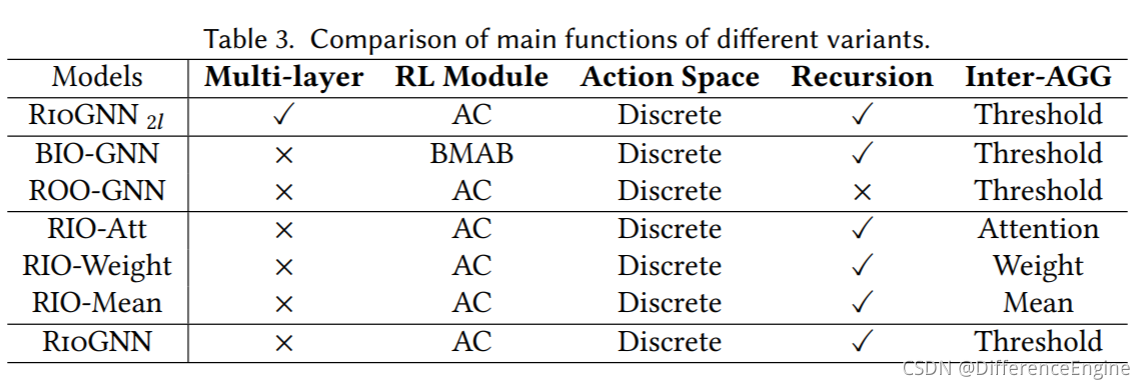• RioGNN 2 l \text{RioGNN}_{2l}：它使用带有离散策略的演员-评论家（AC）算法递归选择不同关系的过滤阈值，并使用过滤阈值作为关系权重来聚合不同关系之间的邻居。 但是标签感知相似性度量使用 2 层结构进行邻居选择。

• BIO-GNN：该变体是一种单层相似性感知邻居选择方法，并使用过滤阈值在关系之间进行聚合。而离散策略的**伯努利多臂老虎机(BMAB) **算法用于递归选择关系的过滤阈值。
• ROO-GNN：该变体是一种具有单层相似性感知邻居选择的方法，并使用过滤阈值进行关系之间的聚合。 但是离散策略的演员-评论家算法用于直接选择关系的过滤阈值。 这种方法可以看作是 RioGNN 的非递归（单深度）版本。

• RIO-Att：该变体使用单层相似度感知进行邻居选择，并使用带有离散策略的演员-评论家算法递归选择不同关系的过滤器阈值。 但是它在聚合不同关系之间的邻居时选择了Attention 的方法。
• RIO-Weight：该变体使用单层相似度感知进行邻居选择，并使用带有离散策略的演员-评论家算法递归选择不同关系的过滤器阈值。 但是它在聚合不同关系之间的邻居时选择了Weight 的方法。
• RIO-Mean：该变体使用单层相似度感知进行邻居选择，并使用带有离散策略的演员-评论家算法递归选择不同关系的过滤器阈值。 但是在聚合不同关系之间的邻居时，它选择了Mean 的方法。

#### 4.3.3 强化学习变体

• AC：Actor-Critic（AC）方法结合了基于价值的方法和基于策略的方法的优点。 采用基于值的方法训练Q函数，提高样本利用效率。 使用基于策略的方法来训练策略，适用于离散和连续的动作空间。 这种方法可以看作是连续动作空间中基于值的方法的扩展，或者是对基于策略的方法的改进，以减少采样方差。
• DQN：深度Q学习（DQN）是一种基于时间差分、基于值的off-policy强化学习方法。DQN通过函数逼近近似地解决了Q-Learning方法在面对高维状态和行为时的维灾难问题。此外，传统的Q-Learning方法使用带有时间序列的样本进行单步更新，并且Q Q值由采样连续性更新。DQN使用随机数据进行梯度下降，这是由于尝试和错误收集了大量样本，这可以打破数据之间的相关性。
• PPO：近端策略优化 (PPO) 在策略梯度 (PG) 的基础上限制更新步长，以防止策略崩溃并使算法上升更稳定。

• AC：我们使用 AC 方法在离散动作空间和连续动作空间中进行变体实验。
• DDPG：Deep Deterministic Policy Gradient (DDPG) 是 DeepMind 开发的一种用于连续控制的 off-policy 算法，比 PPO 具有更高的样本效率。 DDPG 训练确定性策略，即每个状态只考虑一个最优动作。
• SAC：Soft Actor-Critic (SAC) 是一种为最大熵强化学习开发的off-policy算法。 与 DDPG 相比，Soft Actor-Critic 使用随机策略，相对于确定性策略具有一定优势。 Soft Actor-Critic 在公共基准测试中取得了优异的成绩，可以直接应用于真实机器人。
• TD3：Twin Delayed Deep Deterministic policy gradient (TD3) 是一种时间差分、基于策略的策略梯度强化学习方法。 TD3 是 DDPG 的优化版本。 它使用两组网络来估计Q Q值，使用相对较小的一组作为更新目标。

### 4.5 评估指标

Recall  = T P T P + F N , (17) \text { Recall }=\frac{T P}{T P+F N},\tag{17}

A U C = 1 2 ∑ i = 1 m − 1 ( x i + 1 − x i ) ( y i + y i + 1 ) , (18) A U C=\frac{1}{2} \sum_{i=1}^{m-1}\left(x_{i+1}-x_{i}\right)\left(y_{i}+y_{i+1}\right),\tag{18}

A R I = ∑ i j ( n i j 2 ) − [ ∑ i ( a i 2 ) ∑ j ( b j 2 ) ] / ( n 2 ) 1 2 [ ∑ i ( a i 2 ) − ∑ j ( 2 b j ) ] − [ ∑ i ( a i 2 ) ∑ j ( b j 2 ) ] / ( n 2 ) , (19) ARI=\frac{\sum_{i j}\left(\begin{array}{l} n_{i j} \\ 2 \end{array}\right)-\left[\sum_{i}\left(\begin{array}{l} a_{i} \\ 2 \end{array}\right) \sum_{j}\left(\begin{array}{l} b_{j} \\ 2 \end{array}\right)\right] /\left(\begin{array}{l} n \\ 2 \end{array}\right)}{\frac{1}{2}\left[\sum_{i}\left(\begin{array}{c} a_{i} \\ 2 \end{array}\right)-\sum_{j}\left({ }_{2}^{b_{j}}\right)\right]-\left[\sum_{i}\left(\begin{array}{c} a_{i} \\ 2 \end{array}\right) \sum_{j}\left(\begin{array}{c} b_{j} \\ 2 \end{array}\right)\right] /\left(\begin{array}{c} n \\ 2 \end{array}\right)},\tag{19}

N M I = I ( ω ; C ) [ H ( ω ) + H ( C ) ] / 2 , (20) N M I=\frac{I(\omega ; C)}{[H(\omega)+H(C)] / 2},\tag{20}

F 1 = 2 ⋅  Precision  ⋅  Recall   Precision  +  Recal  , (21) F 1=2 \cdot \frac{\text { Precision } \cdot \text { Recall }}{\text { Precision }+\text { Recal }},\tag{21}

## 五、结果与讨论

### 5.1 欺诈检测任务的总体评估

#### 5.1.1 准确性分析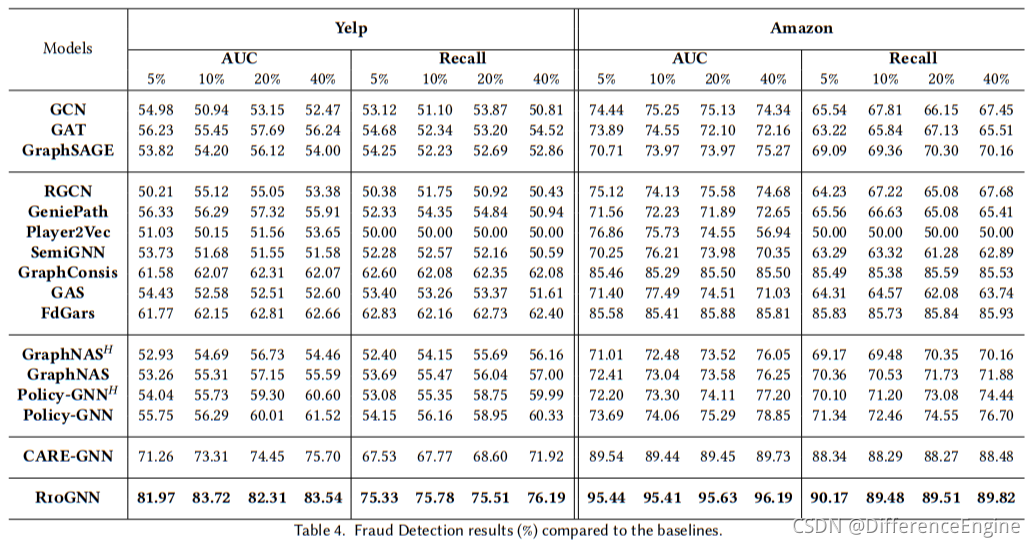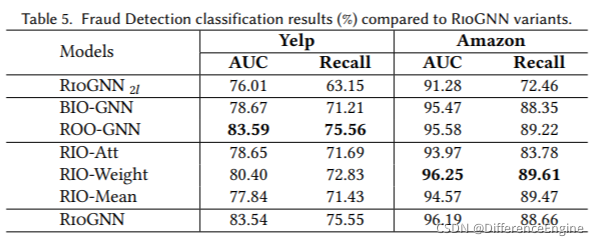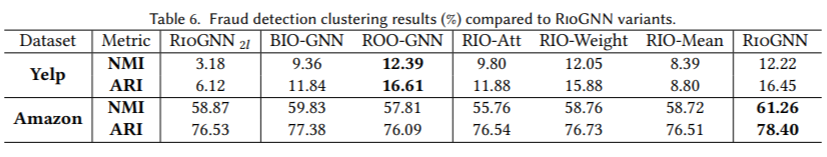#### 5.1.2 可解释的RSRL训练过程。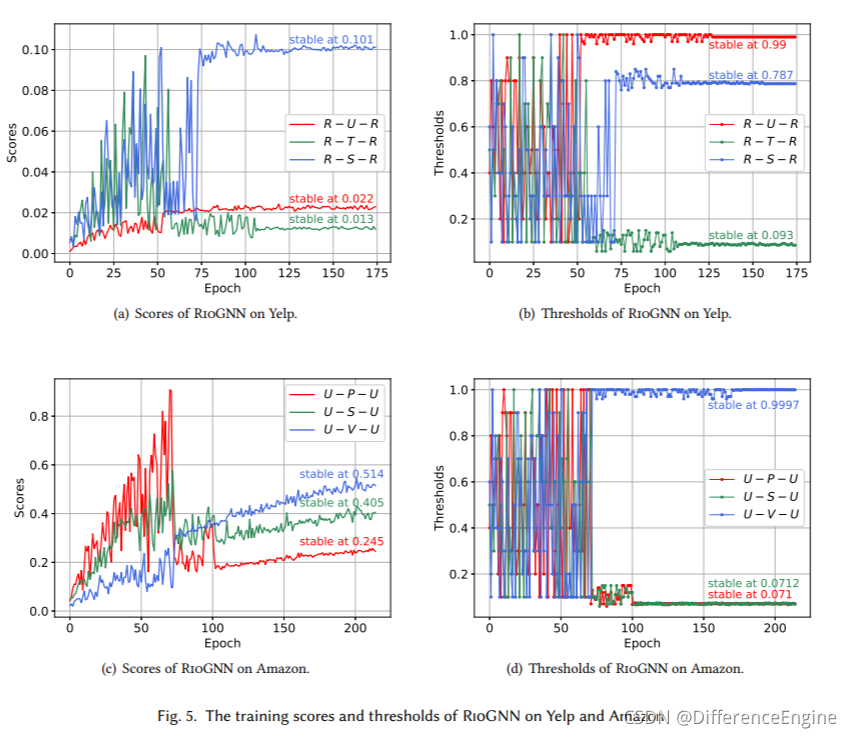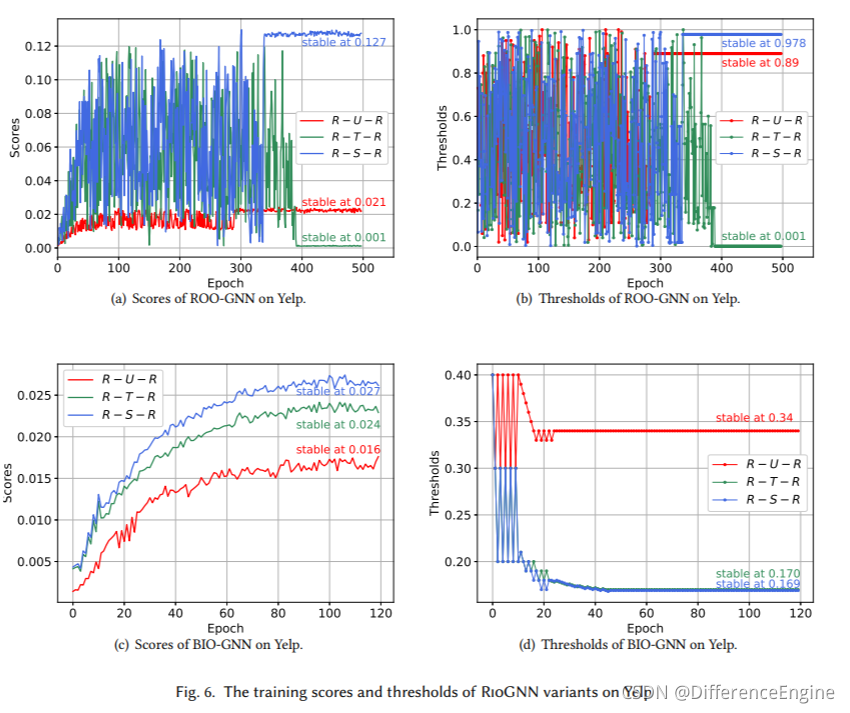#### 5.1.3 效果和效率评价。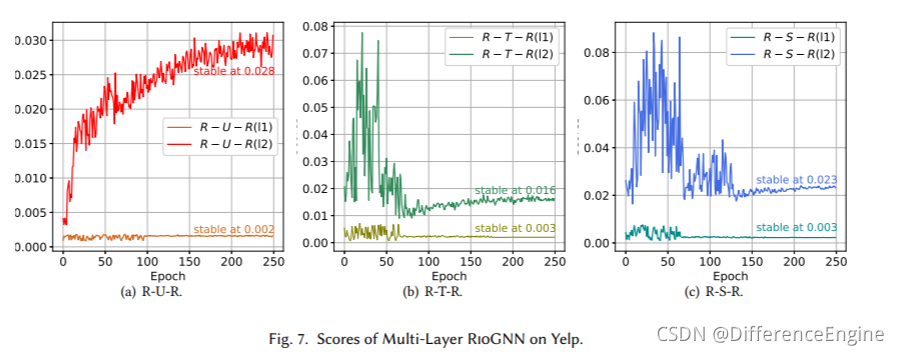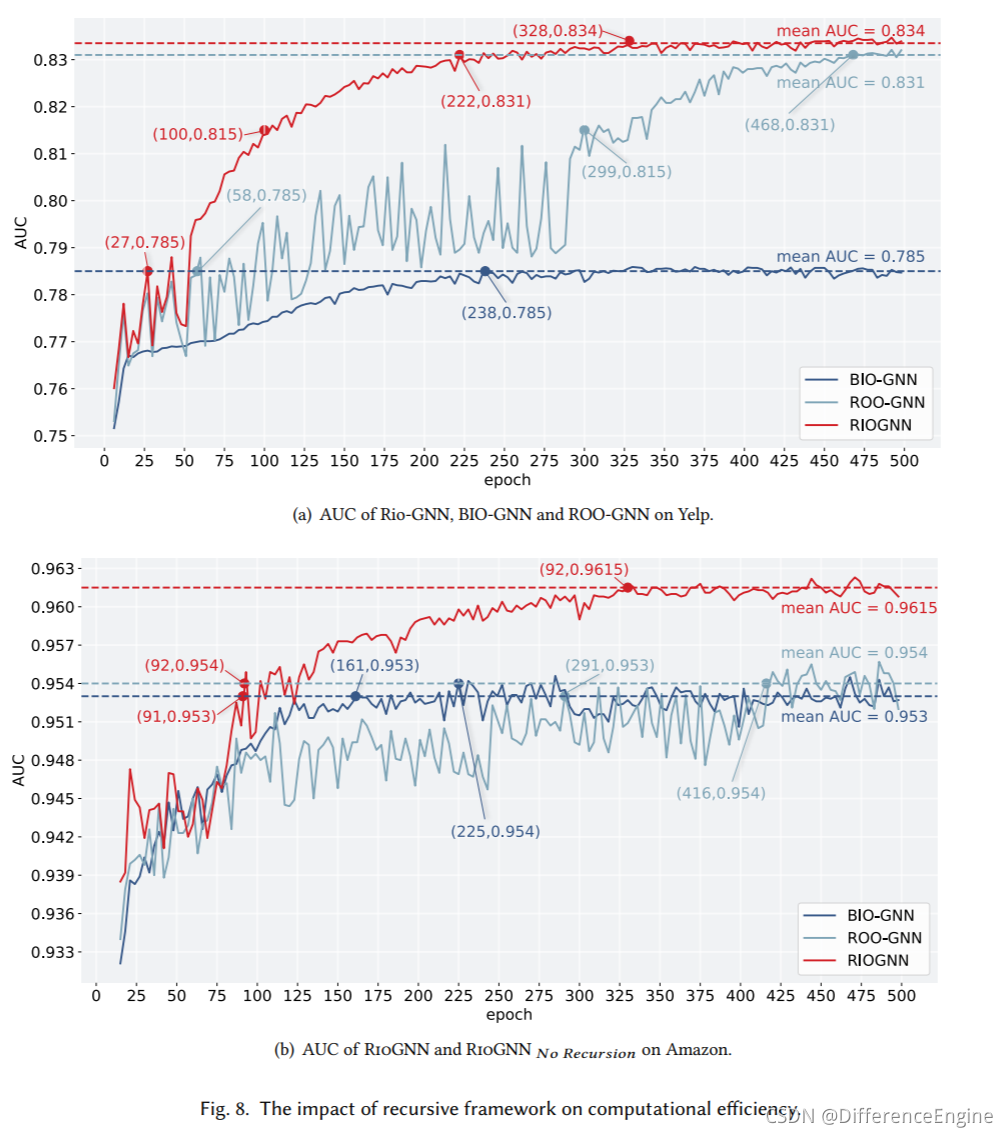### 5.2 糖尿病诊断任务的综合评价

#### 5.2.1 准确性分析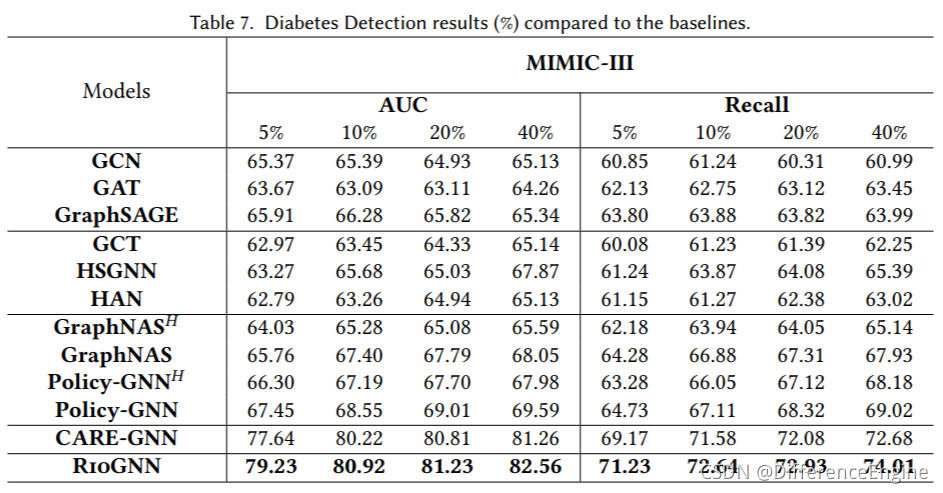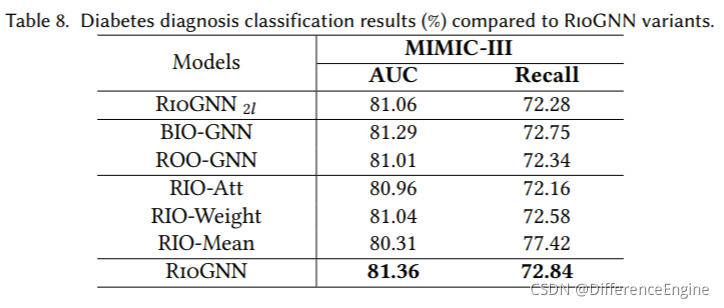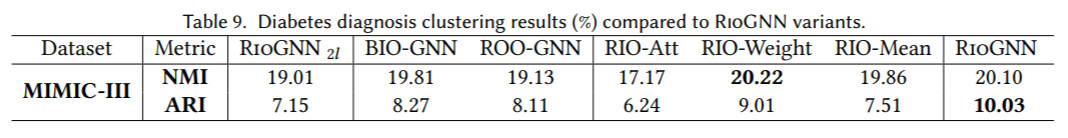#### 5.2.2 可解释的RSRL训练过程。

**RSRL 框架的有效性和必要性。**这部分通过比较 RioGNN 与变体及其初步版本 CARE-GNN 来证明所提出框架的有效性和必要性。 从图 10 可以看出，RioGNN 几乎在每个 epoch 中的表现都优于 CARE-GNN，这证明 RSRL 框架对 MIMIC-III 上的最终分类结果有积极影响。 每个关系并更准确地识别可疑节点。与RioGNN相比，图9中ROO-GNN的准确率波动较大，难以收敛到一个稳定的范围，证明了建立递归过程的必要性。 通过RSRL过程，分类精度可以保持在一个相对稳定的范围内。 实验结果表明，通过对每个深度进行更准确的过滤阈值搜索，具有递归框架的RioGNN表现更好。 而没有递归的 ROO-GNN 不仅无法在有限数量的 epoch 内收敛，而且会导致精度损失。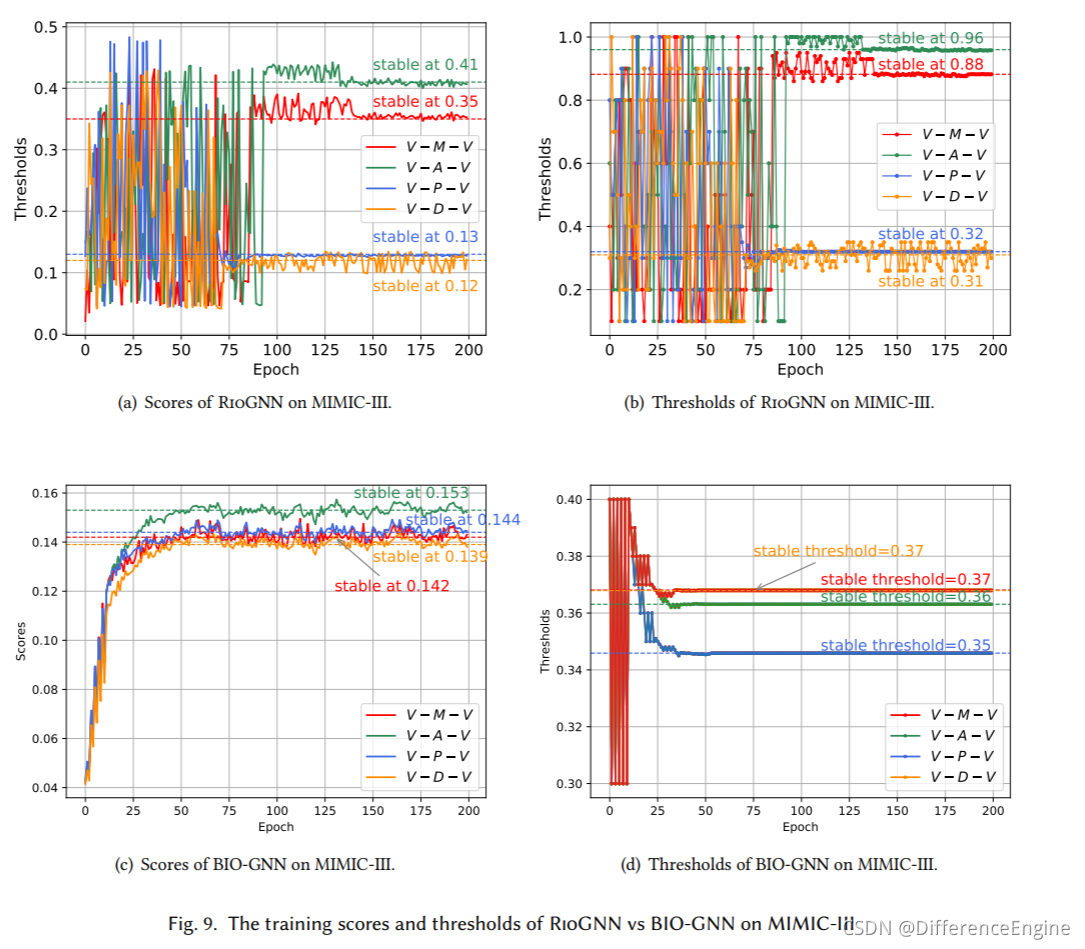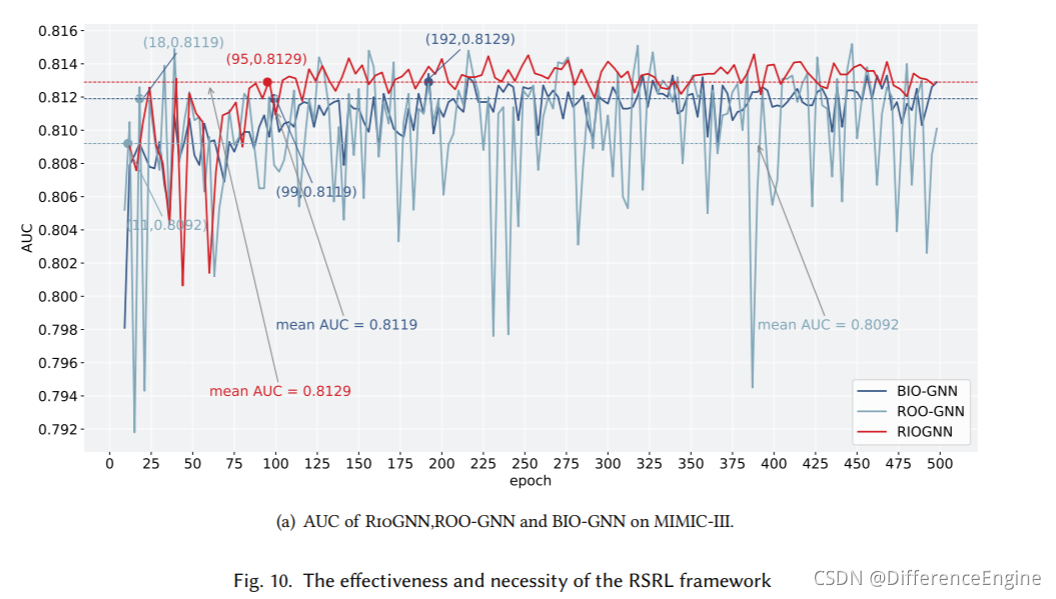### 5.3 RSRL框架的通用性分析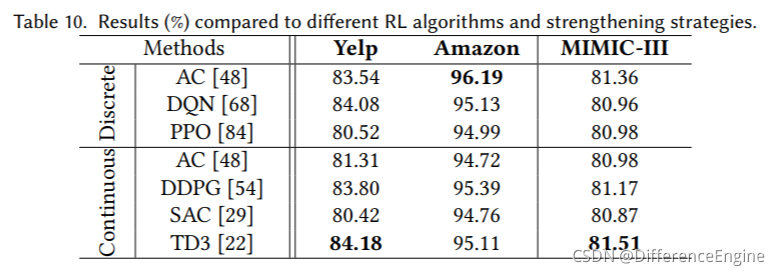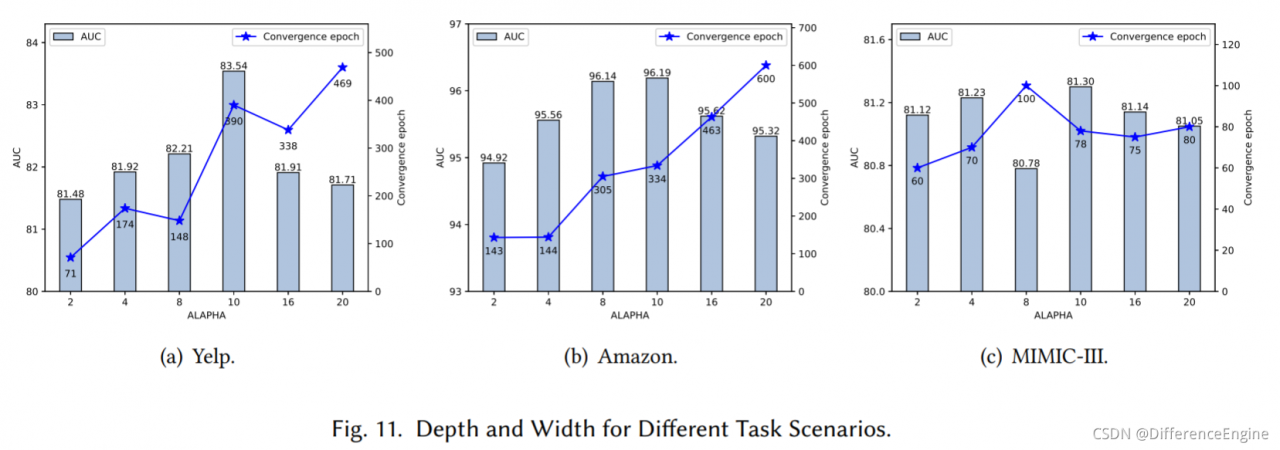### 5.4 归纳学习分析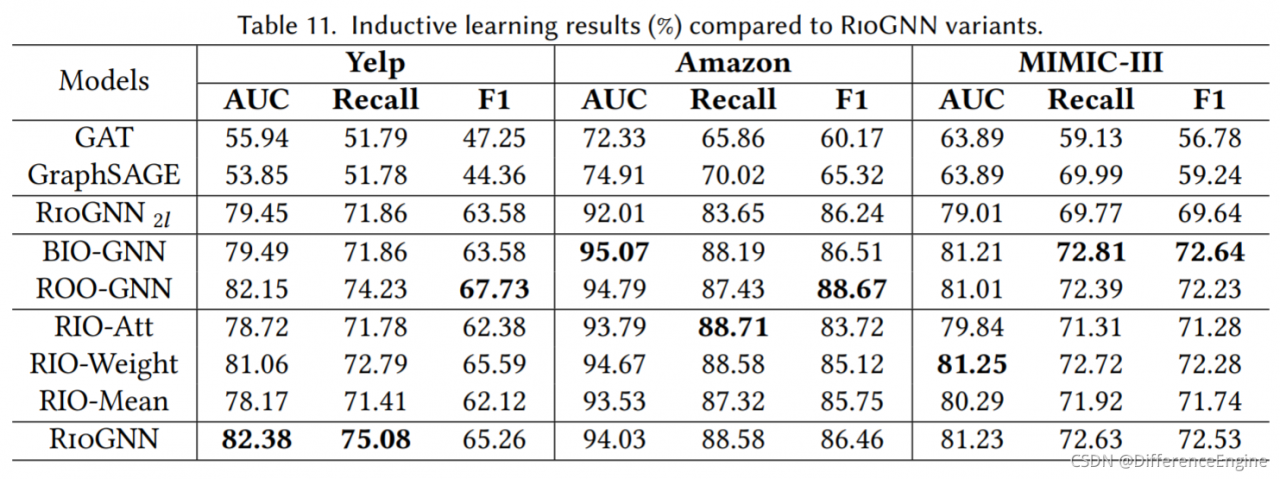### 5.5 超参数灵敏度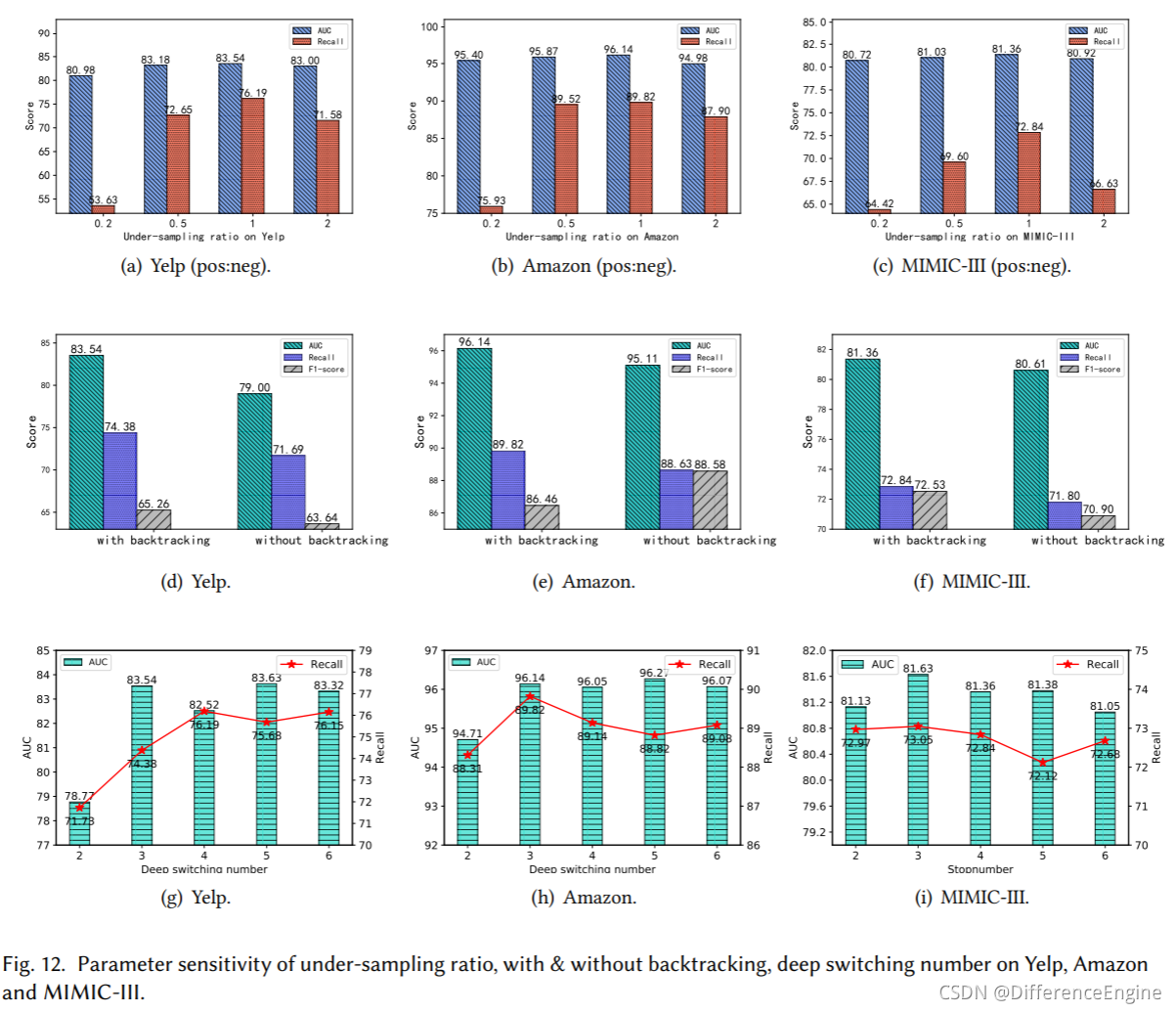## 参考文献

Aisha Abdallah, Mohd Aizaini Maarof, and Anazida Zainal. 2016. Fraud detection system: A survey.Journal of Network and Computer Applications68 (2016), 90–113.

Paul Almasan, José Suárez-Varela, Arnau Badia-Sampera, Krzysztof Rusek, Pere Barlet-Ros, and Albert Cabellos-Aparicio. 2019. Deep reinforcement learning meets graph neural networks: Exploring a routing optimization use case.arXiv preprint arXiv:1910.07421(2019).

Victor Bapst, Thomas Keck, A Grabska-Barwińska, Craig Donner, Ekin Dogus Cubuk, Samuel S Schoenholz, Annette Obika, Alexander WR Nelson, Trevor Back, Demis Hassabis, et al.2020. Unveiling the predictive power of static structure in glassy systems.Nature Physics16, 4 (2020), 448–454.

Smriti Bhagat, Graham Cormode, and S Muthukrishnan. 2011. Node classification in social networks. InSocial network data analytics. Springer, 115–148.

Bokai Cao, Mia Mao, Siim Viidu, and Philip S. Yu. 2017. HitFraud: a broad learning approach for collective fraud detection in heterogeneous information networks. InProceedings of the IEEE ICDM. 769–774.

Yuwei Cao, Hao Peng, and Philip S. Yu. 2020. Multi-information Source HIN for Medical Concept Embedding. InProceedings of the PAKDD. Springer, 396–408.

Hao Chen, Yue Xu, Feiran Huang, Zengde Deng, Wenbing Huang, Senzhang Wang, Peng He, and Zhoujun Li. 2020. Label-Aware Graph Convolutional Networks. InProceedings of the CIKM. ACM, 1977–1980.

Yifan Chen, Pengjie Ren, Yang Wang, and Maarten de Rijke. 2019. Bayesian Personalized Feature Interaction Selection for Factorization Machines. InProceedings of ACM SIGIR. 665–674.

Yifan Chen, Yang Wang, Xiang Zhao, Jie Zou, and Maarten De Rijke. 2020. Block-Aware Item Similarity Models for Top-N Recommendation.ACM Transactions on Information Systems38, 4, Article 42 (2020), 26 pages.

Yu Chen, Lingfei Wu, and Mohammed J Zaki. 2019. Deep iterative and adaptive learning for graph neural networks.arXiv preprint arXiv:1912.07832(2019).

Yu Chen, Lingfei Wu, and Mohammed J Zaki. 2019. Reinforcement Learning Based Graph-to-Sequence Model for Natural Question Generation. InProceedings of the ICLR.

Wei-Lin Chiang, Xuanqing Liu, Si Si, Yang Li, Samy Bengio, and Cho-Jui Hsieh. 2019. Cluster-GCN: An efficient algorithm for training deep and large graph convolutional networks. InProceedings of the SIGKDD. ACM, 257–266.

Edward Choi, Zhen Xu, Yujia Li, Michael Dusenberry, Gerardo Flores, Emily Xue, and Andrew Dai. 2020. Learning the graphical structure of electronic health records with graph convolutional transformer. InProceedings of the AAAI, Vol. 34. 606–613.

Yingtong Dou. 2019. A review of recent advance in online spam detection. (2019).

Yingtong Dou, Zhiwei Liu, Li Sun, Yutong Deng, Hao Peng, and Philip S. Yu. 2020. Enhancing Graph Neural Network-based Fraud
Detectors against Camouflaged Fraudsters. InProceedings of the CIKM. ACM, 315–324.

Yingtong Dou, Guixiang Ma, Philip S. Yu, and Sihong Xie. 2020. Robust Spammer Detection by Nash Reinforcement Learning. In Proceedings of the KDD. ACM, 924–933.

Gabriel Dulac-Arnold, Richard Evans, Hado van Hasselt, Peter Sunehag, Timothy Lillicrap, Jonathan Hunt, Timothy Mann, Theophane Weber, Thomas Degris, and Ben Coppin. 2016. Deep Reinforcement Learning in Large Discrete Action Spaces. arXiv:1512.07679 [cs.AI]

David K Duvenaud, Dougal Maclaurin, Jorge Iparraguirre, Rafael Bombarell, Timothy Hirzel, Alán Aspuru-Guzik, and Ryan P Adams. 2015. Convolutional networks on graphs for learning molecular fingerprints. InProceedings of the NIPS. 2224–2232.

Wenqi Fan, Yao Ma, Qing Li, Yuan He, Eric Zhao, Jiliang Tang, and Dawei Yin. 2019. Graph neural networks for social recommendation. InProceedings of the Web Conference. ACM, 417–426.

Meherwar Fatima, Maruf Pasha, et al.2017. Survey of machine learning algorithms for disease diagnostic.Journal of Intelligent Learning Systems and Applications9, 01 (2017), 1.

Xinyu Fu, Jiani Zhang, Ziqiao Meng, and Irwin King. 2020. MAGNN: Metapath Aggregated Graph Neural Network for Heterogeneous Graph Embedding. InProceedings of the WWW. ACM, 2331–2341.

Scott Fujimoto, Herke van Hoof, and David Meger. 2018. Addressing Function Approximation Error in Actor-Critic Methods. In Proceedings of the 35th International Conference on Machine Learning (Proceedings of Machine Learning Research, Vol. 80). PMLR, Stockholmsmässan, Stockholm Sweden, 1587–1596.

Yang Gao, Hong Yang, Peng Zhang, Chuan Zhou, and Yue Hu. 2019. Graphnas: Graph neural architecture search with reinforcement learning.arXiv preprint arXiv:1904.09981(2019).

Justin Gilmer, Samuel S Schoenholz, Patrick F Riley, Oriol Vinyals, and George E Dahl. 2017. Neural message passing for quantum chemistry. InProceedings of the ICML. PMLR, 1263–1272.

A. L. Goldberger, L. A. N. Amaral, L. Glass, J. M. Hausdorff, P. Ch. Ivanov, R. G. Mark, J. E. Mietus, G. B. Moody, C. K. Peng, and H. E. Stanley. 2000. PhysioBank, PhysioToolkit, and PhysioNet: components of a new research resource for complex physiologic signals. Circulation101, 23 (2000), E215.

Priya Goyal, Piotr Dollár, Ross Girshick, Pieter Noordhuis, Lukasz Wesolowski, Aapo Kyrola, Andrew Tulloch, Yangqing Jia, and Kaiming He. 2017. Accurate, large minibatch sgd: Training imagenet in 1 hour.arXiv preprint arXiv:1706.02677(2017).

Steve Gregory. 2010. Finding overlapping communities in networks by label propagation.New journal of Physics12, 10 (2010), 103018.

Shengnan Guo, Youfang Lin, Ning Feng, Chao Song, and Huaiyu Wan. 2019. Attention based spatial-temporal graph convolutional networks for traffic flow forecasting. InProceedings of the AAAI, Vol. 33. AAAI Press, 922–929.

Tuomas Haarnoja, Aurick Zhou, Kristian Hartikainen, George Tucker, Sehoon Ha, Jie Tan, Vikash Kumar, Henry Zhu, Abhishek Gupta, Pieter Abbeel, et al. 2018. Soft actor-critic algorithms and applications.arXiv preprint arXiv:1812.05905(2018).

Will Hamilton, Zhitao Ying, and Jure Leskovec. 2017. Inductive representation learning on large graphs. InProceedings of the NIPS. 1024–1034.

Bowen Hao, Jing Zhang, Hongzhi Yin, Cuiping Li, and Hong Chen. 2021. Pre-Training Graph Neural Networks for Cold-Start Users and Items Representation. InProceedings of the WSDM. ACM, 265–273.

Patrick Hart and Alois Knoll. 2020. Graph Neural Networks and Reinforcement Learning for Behavior Generation in Semantic Environments. InIEEE Intelligent Vehicles Symposium (IV). IEEE, 1589–1594.

Hado van Hasselt, Arthur Guez, and David Silver. 2016. Deep reinforcement learning with double Q-Learning. InProceedings of the AAAI. AAAI Press, 2094–2100.

Yu He, Yangqiu Song, Jianxin Li, Cheng Ji, Jian Peng, and Hao Peng. 2019. HeteSpaceyWalk: a heterogeneous spacey random walk for heterogeneous information network embedding. InProceedings of the CIKM. ACM, 639–648.

Huiting Hong, Hantao Guo, Yucheng Lin, Xiaoqing Yang, Zang Li, and Jieping Ye. 2020. An attention-based graph neural network for heterogeneous structural learning. InProceedings of the AAAI. 4132–4139.

Anahita Hosseini, Ting Chen, Wenjun Wu, Yizhou Sun, and Majid Sarrafzadeh. 2018. Heteromed: Heterogeneous information network for medical diagnosis. InProceedings of the CIKM. ACM, 763–772. Junling Hu and Michael P. Wellman. 1998. Multiagent Reinforcement Learning: Theoretical Framework and an Algorithm. InProceedings of the Fifteenth International Conference on Machine Learning (ICML ’98). Morgan Kaufmann Publishers Inc., San Francisco, CA, USA, 242–250.

Ziniu Hu, Yuxiao Dong, Kuansan Wang, and Yizhou Sun. 2020. Heterogeneous graph transformer. InProceedings of the WWW. ACM, 2704–2710.

Jaromír Janisch, Tomáš Pevny, and Viliam Lisy. 2020. Symbolic Relational Deep Reinforcement Learning based on Graph Neural Networks.arXiv preprint arXiv:2009.12462(2020).

Di Jin, Ziyang Liu, Weihao Li, Dongxiao He, and Weixiong Zhang. 2019. Graph convolutional networks meet markov random fields: Semi-supervised community detection in attribute networks. InProceedings of the AAAI. AAAI Press, 152–159.

Alistair EW Johnson, Tom J Pollard, Lu Shen, H Lehman Li-Wei, Mengling Feng, Mohammad Ghassemi, Benjamin Moody, Peter Szolovits, Leo Anthony Celi, and Roger G Mark. 2016. MIMIC-III, a freely accessible critical care database.Scientific data3, 1 (2016), 1–9.

Alistair E. W Johnson, Tom J Pollard, Lu Shen, Li Wei H Lehman, Mengling Feng, Mohammad Ghassemi, Benjamin Moody, Peter Szolovits, Leo Anthony Celi, and Roger G Mark. [n.d.]. MIMIC-III, a freely accessible critical care database.Scientific Data([n. d.]).

Feng Jun, Huang Minlie, Zhao Li, Yang Yang, and Zhu Xiaoyan. 2018. Reinforcement Learning for Relation Classification from Noisy Data. arXiv:1808.08013 [cs.IR]

P. Kaghazgaran, J. Caverlee, and A. Squicciarini. 2018. Combating crowdsourced review manipulators: A neighborhood-based approach. InProceedings of the WSDM. ACM, 306–314.

Anssi Kanervisto, Christian Scheller, and Ville Hautamäki. 2020. Action Space Shaping in Deep Reinforcement Learning. In2020 IEEE Conference on Games (CoG). 479–486.

Seyed Mehran Kazemi and David Poole. 2018. Simple embedding for link prediction in knowledge graphs. InProceedings of the NIPS. 4284–4295.

Thomas N Kipf and Max Welling. 2017. Semi-supervised classification with graph convolutional networks.Proceedings of the ICLR.

Vijay R Konda and John N Tsitsiklis. 2000. Actor-critic algorithms. InProceedings of the NIPS. 1008–1014.

Kwei-Herng Lai, Daochen Zha, Kaixiong Zhou, and Xia Hu. 2020. Policy-GNN: Aggregation Optimization for Graph Neural Networks.

Kai Lei, Meng Qin, Bo Bai, Gong Zhang, and Min Yang. 2019. GCN-GAN: A non-linear temporal link prediction model for weighted dynamic networks. InProceedings of the IEEE INFOCOM. 388–396.

Ao Li, Zhou Qin, Runshi Liu, Yiqun Yang, and Dong Li. 2019. Spam review detection with graph convolutional networks. InProceedings of the 28th ACM International Conference on Information and Knowledge Management. ACM, 2703–2711.

Ruoyu Li and Sheng Wang. 2018. Adaptive Graph Convolutional Neural Networks. InProceedings of the AAAI. AAAI Press, 3546–3553.

Ye Li, Chaofeng Sha, Xin Huang, and Yanchun Zhang. 2018. Community detection in attributed graphs: An embedding approach. In Proceedings of the AAAI. AAAI Press, 338–345.

Timothy P. Lillicrap, Jonathan J. Hunt, Alexander Pritzel, Nicolas Heess, Tom Erez, Yuval Tassa, David Silver, and Daan Wierstra. 2019. Continuous control with deep reinforcement learning. arXiv:1509.02971 [cs.LG]

Weiyang Liu, Zhen Liu, James M Rehg, and Le Song. 2019. Neural similarity learning. InProceedings of the NIPS. 5025–5036.

Xin Liu and Tsuyoshi Murata. 2010. Advanced modularity-specialized label propagation algorithm for detecting communities in networks.Physica A: Statistical Mechanics and its Applications389, 7 (2010), 1493–1500.

Ye Liu, Yao Wan, Lifang He, Hao Peng, and Yu Philip S. 2021. KG-BART: Knowledge Graph-Augmented BART for Generative Commonsense Reasoning. InProceedings of the AAAI Conference on Artificial Intelligence, Vol. 35. 6418–6425.

Ziqi Liu, Chaochao Chen, Longfei Li, Jun Zhou, Xiaolong Li, Le Song, and Yuan Qi. 2019. GeniePath: Graph Neural Networks with Adaptive Receptive Paths.Proceedings of the AAAI Conference on Artificial Intelligence33, 01 (Jul. 2019), 4424–4431.

Ziqi Liu, Chaochao Chen, Xinxing Yang, Jun Zhou, Xiaolong Li, and Le Song. 2018. Heterogeneous graph neural networks for malicious account detection. InProceedings of the 27th ACM International Conference on Information and Knowledge Management. 2077–2085.

Zhiwei Liu, Yingtong Dou, Philip S. Yu, Yutong Deng, and Hao Peng. 2020. Alleviating the Inconsistency Problem of Applying Graph Neural Network to Fraud Detection.Proceedings of the SIGIR, 1569–1572.

Zheng Liu, Xiaohan Li, Hao Peng, Lifang He, and Philip S. Yu. 2020. Heterogeneous Similarity Graph Neural Network on Electronic Health Records. InProceedings of the BigData. IEEE.

Chen Lu, Chen Zhi, Tan Bowen, Long Sishan, Gašić Milica, and Yu Kai. 2019. AgentGraph: Toward Universal Dialogue Management With Structured Deep Reinforcement Learning.IEEE/ACM Transactions on Audio, Speech, and Language Processing27, 9 (2019), 1378–1391.

Jake Luo, Christina Eldredge, Chi C Cho, and Ron A Cisler. 2016. Population Analysis of Adverse Events in Different Age Groups Using Big Clinical Trials Data.JMIR Med Inform4, 4 (17 Oct 2016), e30. https://doi.org/10.2196/medinform.6437

Guixiang Ma, Lifang He, Chun-Ta Lu, Weixiang Shao, Philip S. Yu, Alex D Leow, and Ann B Ragin. 2017. Multi-view clustering with graph embedding for connectome analysis. InProceedings of the 2017 ACM on Conference on Information and Knowledge Management. 127–136.

Tengfei Ma, Cao Xiao, Jiayu Zhou, and Fei Wang. 2018. Drug similarity integration through attentive multi-view graph auto-encoders. InProceedings of the 27th International Joint Conference on Artificial Intelligence. 3477–3483.

Julian John McAuley and Jure Leskovec. 2013. From Amateurs to Connoisseurs: Modeling the Evolution of User Expertise through Online Reviews. InProceedings of the 22nd International Conference on World Wide Web(Rio de Janeiro, Brazil)(WWW ’13). Association for Computing Machinery, New York, NY, USA, 897–908.

Changping Meng, Reynold Cheng, Silviu Maniu, Pierre Senellart, and Wangda Zhang. 2015. Discovering meta-paths in large heterogeneous information networks. InProceedings of the WWW. ACM, 754–764.

Volodymyr Mnih, Koray Kavukcuoglu, David Silver, Andrei A Rusu, Joel Veness, Marc G Bellemare, Alex Graves, Martin Riedmiller, Andreas K Fidjeland, Georg Ostrovski, et al.2015. Human-level control through deep reinforcement learning.nature518, 7540 (2015), 529–533.

Arjun Mukherjee, Vivek Venkataraman, Bing Liu, and Natalie Glance. 2013. What Yelp Fake Review Filter Might Be Doing?Proceedings of the International AAAI Conference on Web and Social Media7, 1 (Jun. 2013).

Deepak Nathani, Jatin Chauhan, Charu Sharma, and Manohar Kaul. 2019. Learning Attention-based Embeddings for Relation Prediction in Knowledge Graphs. InProceedings of the ACL. ACL, 4710–4723.

Mark EJ Newman. 2004. Detecting community structure in networks.The European physical journal B38, 2 (2004), 321–330.

Shirui Pan, Ruiqi Hu, Guodong Long, Jing Jiang, Lina Yao, and Chengqi Zhang. 2018. Adversarially regularized graph autoencoder for graph embedding. InProceedings of the IJCAI. AAAI Press, 2609–2615.

Hao Peng, Jianxin Li, Qiran Gong, Yuanxin Ning, Senzhang Wang, and Lifang He. 2020. Motif-Matching Based Subgraph-Level Attentional Convolutional Network for Graph Classification. InProceedings of the AAAI Conference on Artificial Intelligence, Vol. 34. 5387–5394.

Hao Peng, Jianxin Li, Qiran Gong, Yangqiu Song, Yuanxing Ning, Kunfeng Lai, and Philip S. Yu. 2019. Fine-grained event categorization with heterogeneous graph convolutional networks. InProceedings of the IJCAI. AAAI Press, 3238–3245.

Hao Peng, Jianxin Li, Yu He, Yaopeng Liu, Mengjiao Bao, Lihong Wang, Yangqiu Song, and Qiang Yang. 2018. Large-scale hierarchical text classification with recursively regularized deep graph-cnn. InProceedings of the WWW. 1063–1072.

Hao Peng, Jianxin Li, Yangqiu Song, Renyu Yang, Ranjan Rajiv, Philip S. Yu, and He Lifang. 2021. Streaming Social Event Detection and Evolution Discovery in Heterogeneous Information Networks.ACM Transactions on Knowledge Discovery from Data(2021).

Hao Peng, Jianxin Li, Senzhang Wang, Lihong Wang, Qiran Gong, Renyu Yang, Bo Li, Philip S. Yu, and Lifang He. 2019. Hierarchical taxonomy-aware and attentional graph capsule RCNNs for large-scale multi-label text classification.IEEE Transactions on Knowledge and Data Engineering(2019).

Hao Peng, Hongfei Wang, Bowen Du, Md Zakirul Alam Bhuiyan, Hongyuan Ma, Jianwei Liu, Lihong Wang, Zeyu Yang, Linfeng Du, Senzhang Wang, et al.2020. Spatial temporal incidence dynamic graph neural networks for traffic flow forecasting.Information Sciences521 (2020), 277–290.

D. Pozo and J. Contreras. 2011. Finding Multiple Nash Equilibria in Pool-Based Markets: A Stochastic EPEC Approach.IEEE Transactions on Power Systems26, 3 (2011), 1744–1752.

Ruihong Qiu, Zi Huang, Jingjing Li, and Hongzhi Yin. 2020. Exploiting Cross-session Information for Session-based Recommendation with Graph Neural Networks.ACM Transactions on Information Systems (TOIS)38, 3 (2020), 1–23.

Usha Nandini Raghavan, Réka Albert, and Soundar Kumara. 2007. Near linear time algorithm to detect community structures in large-scale networks.Physical review E76, 3 (2007), 036106.

Shebuti Rayana and Leman Akoglu. 2015. Collective opinion spam detection: Bridging review networks and metadata. InProceedings of the SIGKDD. ACM, 985–994.

Michael Schlichtkrull, Thomas N Kipf, Peter Bloem, Rianne Van Den Berg, Ivan Titov, and Max Welling. 2018. Modeling relational data with graph convolutional networks. InProceedings of the ESWC. Springer, 593–607.

John Schulman, Filip Wolski, Prafulla Dhariwal, Alec Radford, and Oleg Klimov. 2017. Proximal policy optimization algorithms.arXiv preprint arXiv:1707.06347(2017).

Chuan Shi, Yitong Li, Jiawei Zhang, Yizhou Sun, and S. Yu Philip. 2016. A survey of heterogeneous information network analysis.IEEE Transactions on Knowledge and Data Engineering29, 1 (2016), 17–37.

Junkai Sun, Junbo Zhang, Qiaofei Li, Xiuwen Yi, Yuxuan Liang, and Yu Zheng. 2020. Predicting citywide crowd flows in irregular regions using multi-view graph convolutional networks.IEEE Transactions on Knowledge and Data Engineering(2020).

Lichao Sun, Yingtong Dou, Carl Yang, Ji Wang, Philip S. Yu, Lifang He, and Bo Li. 2018. Adversarial Attack and Defense on Graph Data: A Survey.arXiv preprint arXiv:1812.10528(2018).

Penghao Sun, Julong Lan, Junfei Li, Zehua Guo, and Yuxiang Hu. 2020. Combining Deep Reinforcement Learning With Graph Neural Networks for Optimal VNF Placement.IEEE Communications Letters(2020).

Qingyun Sun, Hao Peng, Jianxin Li, Senzhang Wang, Xiangyu Dong, Liangxuan Zhao, Philip S. Yu, and Lifang He. 2020. Pairwise Learning for Name Disambiguation in Large-Scale Heterogeneous Academic Networks. (2020), 511–520.

Qingyun Sun, Hao Peng, Jianxin Li, Jia Wu, Yuanxing Ning, Phillip S. Yu, and Lifang He. 2021. SUGAR: Subgraph Neural Network with Reinforcement Pooling and Self-Supervised Mutual Information Mechanism. InProceedings of the Web Conference. 2081–2091.

Yingcheng Sun and Kenneth Loparo. 2019. Opinion spam detection based on heterogeneous information network. InProceedings of the IEEE ICTAI. 1156–1163.

Richard S Sutton and Andrew G Barto. 2018.Reinforcement learning: An introduction. MIT press.

Fawcett Tom. 2006. An introduction to ROC analysis.Pattern Recognition Letters27, 8 (2006), 861–874.

Petar Veličković, Guillem Cucurull, Arantxa Casanova, Adriana Romero, Pietro Lio, and Yoshua Bengio. 2018. Graph attention networks. Proceedings of the ICLR.

Vikas Verma, Meng Qu, Alex Lamb, Yoshua Bengio, Juho Kannala, and Jian Tang. 2019. Graphmix: Regularized training of graph neural networks for semi-supervised learning.arXiv preprint arXiv:1909.11715(2019).

Nikil Wale, Ian A Watson, and George Karypis. 2008. Comparison of descriptor spaces for chemical compound retrieval and classification. Knowledge and Information Systems14, 3 (2008), 347–375.

Daixin Wang, Jianbin Lin, Peng Cui, Quanhui Jia, Zhen Wang, Yanming Fang, Quan Yu, Jun Zhou, Shuang Yang, and Yuan Qi. 2019. A Semi-supervised Graph Attentive Network for Financial Fraud Detection. InProceedings of the IEEE ICDM. 598–607.

Jianyu Wang, Rui Wen, Chunming Wu, Yu Huang, and Jian Xion. 2019. Fdgars: Fraudster detection via graph convolutional networks in online app review system. InProceedings of the World Wide Web Conference. 310–316.

Xiao Wang, Deyu Bo, Chuan Shi, Shaohua Fan, Yanfang Ye, and Philip S. Yu. 2020. A Survey on Heterogeneous Graph Embedding: Methods, Techniques, Applications and Sources.arXiv preprint arXiv:2011.14867(2020).

Xiao Wang, Houye Ji, Chuan Shi, et al.2019. Heterogeneous graph attention network. InProceedings of the Web Conference. ACM, 2022–2032.

Yang Wang. 2021. Survey on Deep Multi-Modal Data Analytics: Collaboration, Rivalry, and Fusion.ACM Transactions on Multimedia Computing, Communications, and Applications17, 1s, Article 10 (2021), 25 pages.

Christopher JCH Watkins and Peter Dayan. 1992. Q-learning.Machine learning8, 3-4 (1992), 279–292.

Zonghan Wu, Shirui Pan, Fengwen Chen, Guodong Long, Chengqi Zhang, and S. Yu Philip. 2020. A comprehensive survey on graph neural networks.IEEE Transactions on Neural Networks and Learning Systems(2020).

Yu Xie, Yuanqiao Zhang, Maoguo Gong, Zedong Tang, and Chao Han. 2020. Mgat: Multi-view graph attention networks.Neural Networks132 (2020), 180–189.

Wenhan Xiong, Thien Hoang, and William Yang Wang. 2017. DeepPath: A Reinforcement Learning Method for Knowledge Graph Reasoning. InProceedings of the EMNLP. ACL, 564–573.

Keyulu Xu, Weihua Hu, Jure Leskovec, and Stefanie Jegelka. 2018. How Powerful are Graph Neural Networks?. InProceedings of the ICLR.

Carl Yang, Yichen Feng, Pan Li, Yu Shi, and Jiawei Han. 2018. Meta-graph based hin spectral embedding: Methods, analyses, and insights. InProceedings of the IEEE ICDM. 657–666.

Selim F Yilmaz and Suleyman S Kozat. 2020. Unsupervised Anomaly Detection via Deep Metric Learning with End-to-End Optimization. arXiv preprint arXiv:2005.05865(2020).

Zhitao Ying, Jiaxuan You, Christopher Morris, Xiang Ren, Will Hamilton, and Jure Leskovec. 2018. Hierarchical graph representation learning with differentiable pooling. InProceedings of the NIPS. 4800–4810.

Chen Yu, Wu Lingfei, and J. Zaki Mohammed. 2020. Deep Iterative and Adaptive Learning for Graph Neural Networks.AAAI Workshops (2020).

Baichuan Zhang and Mohammad Al Hasan. 2017. Name disambiguation in anonymized graphs using network embedding. InProceedings of the CIKM. ACM, 1239–1248.

Chuxu Zhang, Dongjin Song, Chao Huang, Ananthram Swami, and Nitesh V Chawla. 2019. Heterogeneous graph neural network. In Proceedings of the SIGKDD. ACM, 793–803.

Muhan Zhang and Yixin Chen. 2018. Link prediction based on graph neural networks. InProceedings of the NIPS. 5165–5175.

Qingheng Zhang, Zequn Sun, Wei Hu, Muhao Chen, Lingbing Guo, and Yuzhong Qu. 2019. Multi-view Knowledge Graph Embedding for Entity Alignment. InProceedings of the IJCAI. 5429–5435.

Shijie Zhang, Hongzhi Yin, Tong Chen, Quoc Viet Nguyen Hung, Zi Huang, and Lizhen Cui. 2020.GCN-Based User Representation Learning for Unifying Robust Recommendation and Fraudster Detection. Association for Computing Machinery, New York, NY, USA, 689–698.

Xi Zhang, Lifang He, Kun Chen, Yuan Luo, Jiayu Zhou, and Fei Wang. 2018. Multi-view graph convolutional network and its applications on neuroimage analysis for parkinson’s disease. InAMIA Annual Symposium Proceedings, Vol. 2018. 1147.

Yiming Zhang, Yujie Fan, Yanfang Ye, Liang Zhao, and Chuan Shi. 2019. Key Player Identification in Underground Forums over Attributed Heterogeneous Information Network Embedding Framework. InProceedings of the CIKM. ACM, 549–558.

Ziwei Zhang, Peng Cui, and Wenwu Zhu. 2020. Deep learning on graphs: A survey.IEEE Transactions on Knowledge and Data Engineering(2020). H. Zheng, M. Xue, H. Lu, S. Hao, H. Zhu, X. Liang, and K. Ross. 2018. Smoke screener or straight shooter: Detecting elite sybil attacks in user-review social networks.Proceedings of the NDSS.

Zhiqiang Zhong, Cheng-Te Li, and Jun Pang. 2020. Reinforcement Learning Enhanced Heterogeneous Graph Neural Network.arXiv preprint arXiv:2010.13735(2020).

Shichao Zhu, Chuan Zhou, Shirui Pan, Xingquan Zhu, and Bin Wang. 2019. Relation structure-aware heterogeneous graph neural network. InProceedings of the IEEE ICDM. 1534–1539.

Xiaojin Zhu and Zoubin Ghahramani. 2002. Learning from labeled and unlabeled data with label propagation. (2002).

Marinka Zitnik and Jure Leskovec. 2017. Predicting multicellular function through multi-layer tissue networks.Bioinformatics33, 14 (2017), i190–i198.
d K. Ross. 2018. Smoke screener or straight shooter: Detecting elite sybil attacks in user-review social networks.Proceedings of the NDSS.

Zhiqiang Zhong, Cheng-Te Li, and Jun Pang. 2020. Reinforcement Learning Enhanced Heterogeneous Graph Neural Network.arXiv preprint arXiv:2010.13735(2020).

Shichao Zhu, Chuan Zhou, Shirui Pan, Xingquan Zhu, and Bin Wang. 2019. Relation structure-aware heterogeneous graph neural network. InProceedings of the IEEE ICDM. 1534–1539.

Xiaojin Zhu and Zoubin Ghahramani. 2002. Learning from labeled and unlabeled data with label propagation. (2002).

Marinka Zitnik and Jure Leskovec. 2017. Predicting multicellular function through multi-layer tissue networks.Bioinformatics33, 14 (2017), i190–i198.